# Contracting-mapping principle

contractive-mapping principle, contraction-mapping principle

A theorem asserting the existence and uniqueness of a fixed point of a mappingof a complete metric space(or a closed subset of such a space) into itself, if for any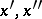the inequality(1)

holds, for some fixed,. This principle is widely used in the proof of the existence and uniqueness of solutions not only of equations of the form, but also of equations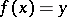, by changing the equation to the equivalent:, where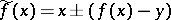.

The scheme of application of the principle is usually as follows: Starting from the properties offirst find a closed set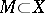, usually a closed ball, such that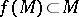, and then prove that on this setis a contractive mapping. After this, starting from an arbitrary element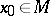, construct the sequence,,belonging to, which converges to some element. This will be the unique solution of the equation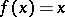, andwill be a sequence of approximate solutions.

In general, condition (1) cannot be changed to(2)

However, if this condition is satisfied on a compact setthat is mapped into itself by, then it guarantees the existence of a unique fixed point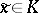for.

The following generalization of the contractive-mapping principle holds. Again, letmap a complete metric spaceinto itself and let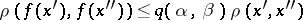for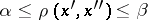, wherefor. Thenhas a unique fixed point in.

How to Cite This Entry:
Contracting-mapping principle. V.I. Sobolev (originator), Encyclopedia of Mathematics. URL: http://encyclopediaofmath.org/index.php?title=Contracting-mapping_principle&oldid=12147
This text originally appeared in Encyclopedia of Mathematics - ISBN 1402006098